# 基于fpga的直流电机vhdl+论文+开题报告+（csdn免费下载）

17 篇文章 6 订阅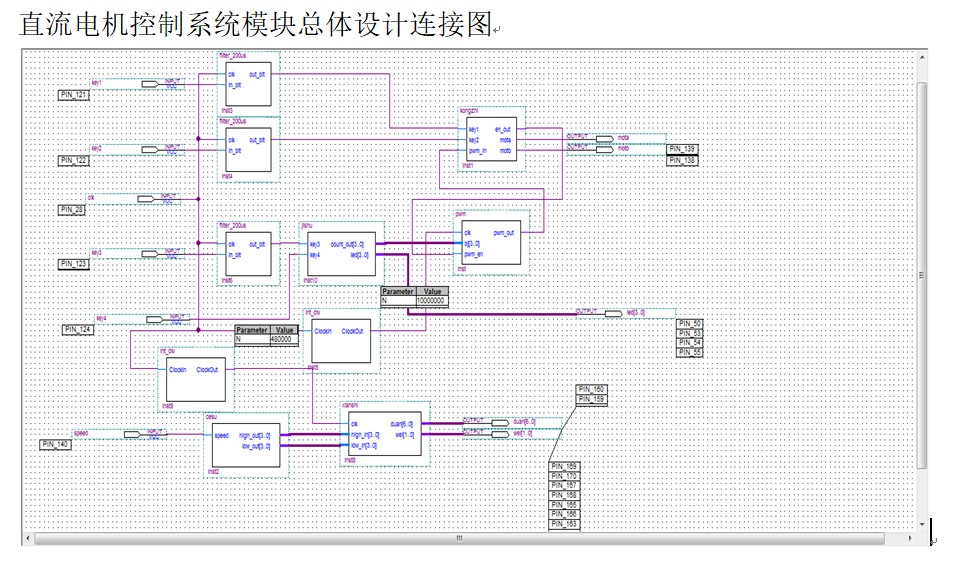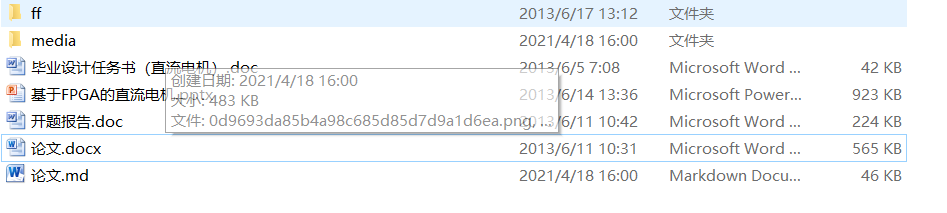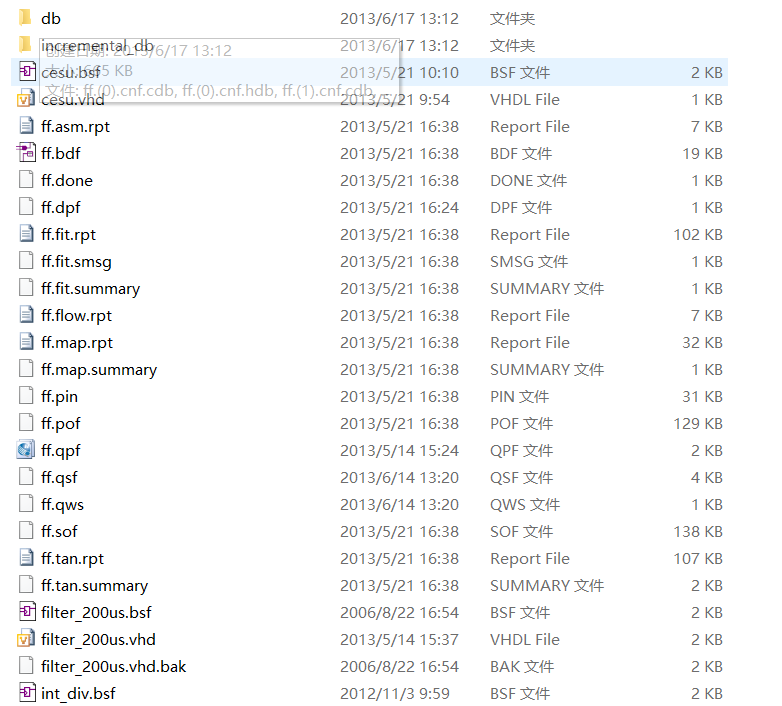# 1 设计软件及开发平台

## 1.1 QuartusII软件简介

Quartus II

Hardware Description
Language）等多种设计输入形式，内嵌自有的综合器以及仿真器，可以完成从设计输入到硬件配置的完整PLD设计流程。而且，Quartus
II提供了完善的用户图形界面设计方式。具有运行速度快，界面统一，功能集中，易学易用等特点。

QuartusII软件支持多种语言，本次设计采用VHDL语言。它是超高速集成电路硬件描述语一言(Very-High-speed
Integrated Cireuit Hardware Description
Language)的缩写,于1985年正式推出,是目前标准化程度最高的硬件描述语一言,IEEE于1987年将VHDL采纳为IEEE#1076标准。经过十几年的发展、应用和完善,VHDL以其强大的系统描述能力、规范的程序设计结构、灵活的语言表达风格和多层次的仿真测试手段,在电子设计领域得到了普遍的认同和广泛的接受,成为现代EDA领域的首选硬件描述语言。目前,流行的EDA工具软件全部支持VHDL,它在EDA领域的学术交流、电子设计的存档、专用集成电路(ASIC)和SOC设计等方面,

## 1.2 SmartEDA实验开发平台

SmartEDA实验开发平台拥有实验开发所需的必须功能，除了核心芯片外，拥有种类丰富、功能强大的外围电路。使用方便快捷，易于操作。其主要功能特点如下：

• 核心板包括用户扩展PACK、4个独立按键、8个LED以及5V电源插座，在PACK上扩展设计后可直接用于用户产品设计和电子设计大赛。

• 采用核心板加主板的灵活结构，核心板可以采用各公司FPGA的各种型号。

• 大部分实验不需要跳线设置，免去了实验前繁琐的跳线设置，实验开发简单快捷。

• 核心板上I/O口PACK考虑的高速特性，可用于高速扩展外设。

• 具有SD卡接口，支持SD、MMC卡读写。

• 8个独立LED灯，8个独立按键，1个蜂鸣器。

• 8个七段数码管。

• 直流电机驱动电路（包括测速电路），可做直流电机闭环调试控制实验。

• 大量实验程序，详细配套教材。

本次设计所用的FPGA为Altera公司Cyclone系列的EP1C6Q240，它兼容EP1C12Q240。EP1C6Q240包含有5980个逻辑单元和92Kbit的片上RAM，拥有185个用户I/O口。

Cyclone
FPGA常用的配置方式有主动配置（AS）、被动配置（PS）以及JTAG配置。Cyclone
FPGA是第一款支持对配置数据进行压缩的FPGA，这使得用户可以将压缩的配置数据存储到配置器件或其他存储器中。

图1-1为SmartEDA实验开发平台的核心电路图。图1-1 SmartEDA实验开发平台的核心电路图

Figure 1-1 the core circuit diagram of SmartEDA

# 2 控制原理

## 2.1 直流电机基本工作原理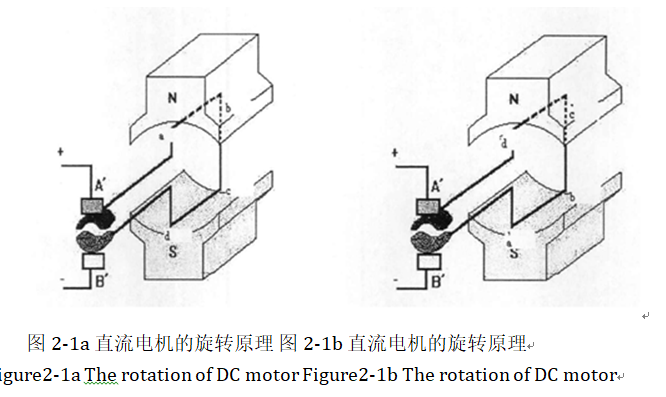Figure2-1a The rotation of DC motor Figure2-1b The rotation of DC motor

## 2.2 直流电机PWM调速原理

### 2.2.1 PWM简介

PWM，即“Pulse Width
Modulation”的英文缩写，其意为脉冲宽度调制。控制方式就是对逆变电路开关器件的通断进行控制，使输出端得到一系列幅值相等的脉冲,这种脉冲被称为PWM

### 2.2.2 PWM调速原理

PWM为一种等幅、占空比可变的方波，改变占空比，即可改变一个周期内电枢两端的平均电压。设占空比为α，周期为T，高电平时间为t1，低电平时间为t2，电枢两端最大电压为U，平均电压为U0，则可得到如下关系

T=t1+t2 (2.1)

α= (2.2)

(2.3)

（1）定宽调频法。T1保持不变,只改变t2,周期T(或频率f)也随之改变。

（2）调宽调频法。T2保持不变,只改变t1,周期T(或频率f)也随之改变。

（3）定频调宽法。周期T(或频率f)保持不变,同时改变t1和t2。

PWM的频率与机械固有频率接近时，将引起震荡。因此，现在多采用第三种方法。

## 2.3 实验箱直流电机电路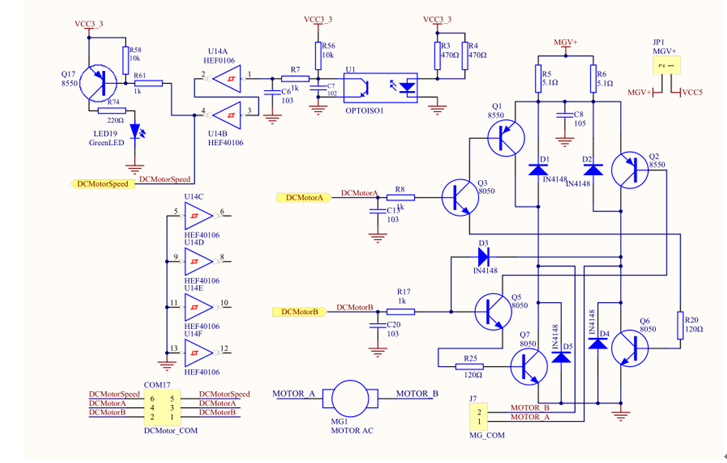Figure 2-2 The circuit of DC motor and tachometer circuit

# 3 控制系统软件设计

## 3.1 总体功能简述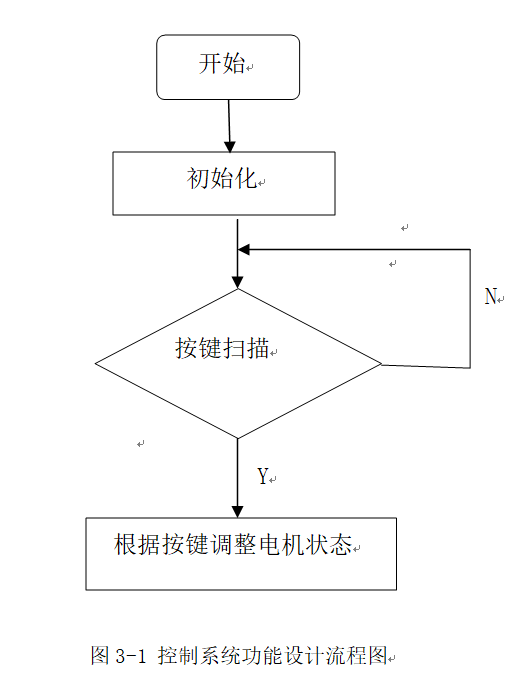Figure 3-1 the flow chart of control system function design

## 3.2 程序逻辑原理

### 3.2.3 显示电路原理

[外链图片转存失败,源站可能有防盗链机制,建议将图片保存下来直接上传(img-pbPDy4Yl-1618936139694)(media/814b54f3b6e875008105811d7cf727eb.png)]
[外链图片转存失败,源站可能有防盗链机制,建议将图片保存下来直接上传(img-3eTIbqdT-1618936139695)(media/0d9693da85b4a98c685d85d7d9a1d6ea.png)]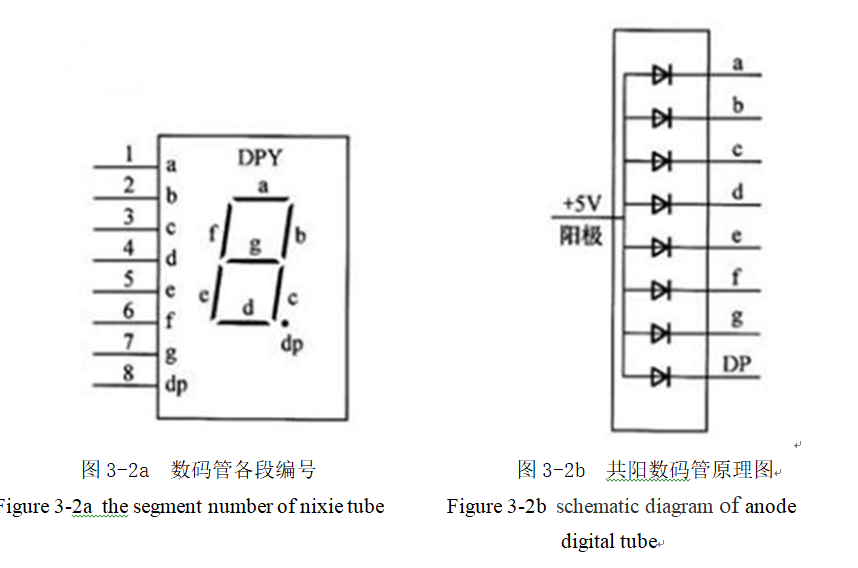Figure 3-2a the segment number of nixie tube Figure 3-2b schematic diagram
of anode digital tube

Table 3-1 the comparison table of LED display character and segment code

0C0H3FH
1F9H06H
2A4H5BH
3B0H4FH
499H66H
592H6DH
682H7DH
7F8H07H
880H7FH
990H6FH

## 3.3 各模块功能设计与分析

### 3.3.1 PWM信号发生模块

PWM信号是整个电机控制系统的基础，没有PWM信号，就无法正常实现对电机的正常控制，因此PWM模块设计的好坏会直接影响设计的最终效果能否让人满意。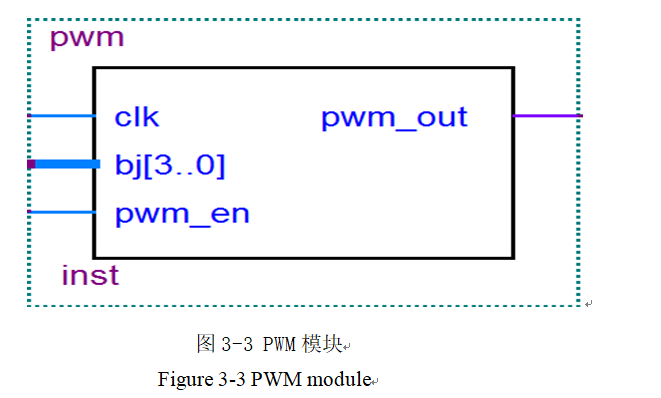Figure 3-3 PWM module

PWM模块程序如下：

library ieee;

use ieee.std_logic_1164.all;

use ieee.std_logic_unsigned.all;

entity pwm is

port(clk:in std_logic;

bj:in std_logic_vector(3 downto 0);

pwm_en:in std_logic;

pwm_out:out std_logic

);

end pwm;

architecture a1 of pwm is

signal cnt:std_logic_vector(3 downto 0);

signal pwm_inside:std_logic;

begin

process(clk,pwm_en)

begin

if(clk’event and clk=‘1’) then

cnt<=cnt+1;

end if;

if(cnt<bj) then

pwm_inside<=‘1’;

else pwm_inside<=‘0’;

end if;

pwm_out<=pwm_inside and pwm_en;

end process;

end;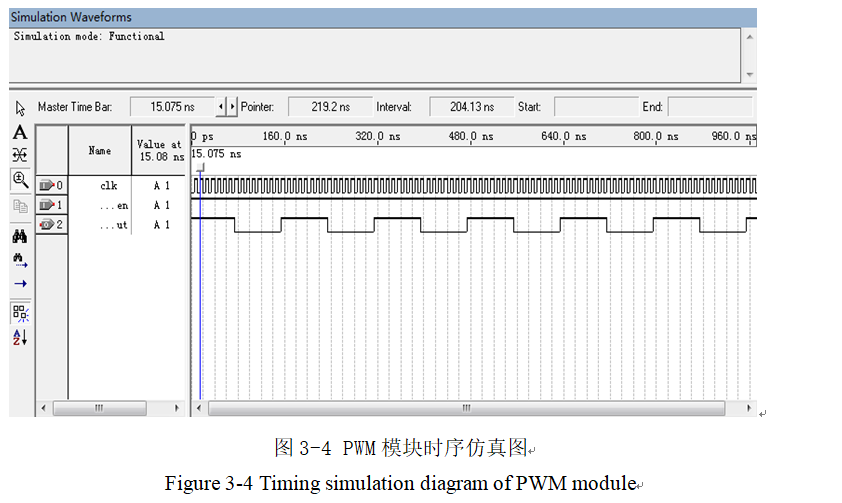Figure 3-4 Timing simulation diagram of PWM module

### 3.3.2 按键计数模块

[外链图片转存失败,源站可能有防盗链机制,建议将图片保存下来直接上传(img-mCCyBKiL-1618936139696)(media/bc2d7a89a63fe12e197c41b7efb530f9.png)]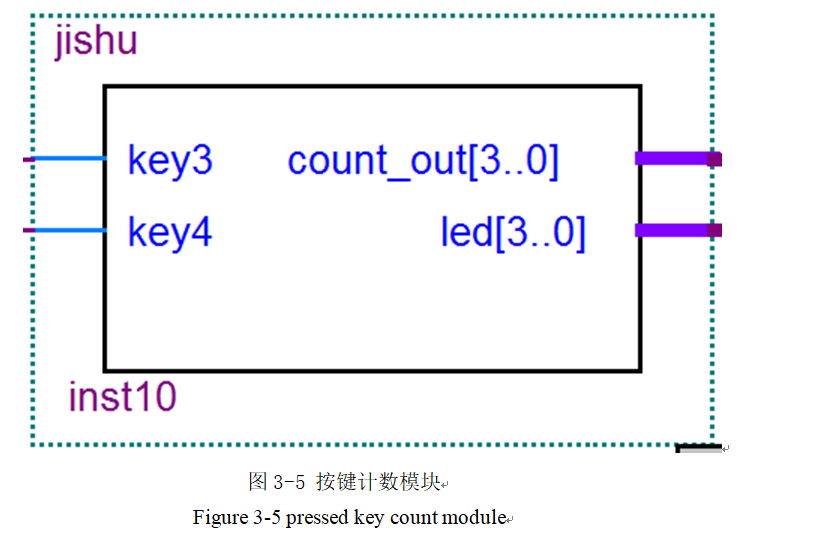Figure 3-5 pressed key count module

library ieee;

use ieee.std_logic_1164.all;

use ieee.std_logic_unsigned.all;

entity jishu is

port(key3,key4:in std_logic;

count_out:out std_logic_vector(3 downto 0);

led:out std_logic_vector(3 downto 0)

);

end jishu;

architecture aa of jishu is

begin

process(key3)

variable cnt:std_logic_vector(3 downto 0);

variable de:std_logic_vector(3 downto 0);

begin

if(key4=‘0’) then

de:=“1111”;

else

de:=“0001”;

end if;

if(key3’event and key3=‘0’) then

cnt:=cnt+de;

end if;

count_out<=cnt;

led<=cnt;

end process;

end;

### 3.3.3 主控模块

[外链图片转存失败,源站可能有防盗链机制,建议将图片保存下来直接上传(img-WA4c7rIj-1618936139698)(media/6e85ab2a98ea244dbf101075f33904df.png)]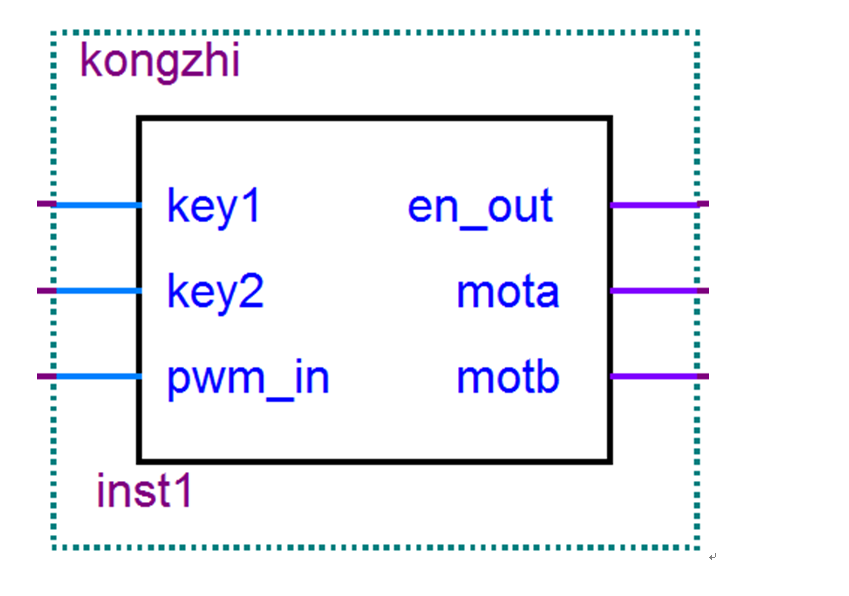Figure 3-6 master control module

library ieee;

use ieee.std_logic_1164.all;

use ieee.std_logic_unsigned.all;

entity kongzhi is

port(key1,key2:in std_logic;

pwm_in:in std_logic;

en_out:out std_logic;

mota:out std_logic;

motb:out std_logic

);

end kongzhi;

architecture a2 of kongzhi is

signal z_f:std_logic;

signal q_t:std_logic;

begin

process(key1,key2)

variable count:std_logic_vector(3 downto 0);

begin

if(key1=‘0’) then

q_t<=not q_t;

end if;

if(key2=‘0’) then

z_f<=not z_f;

end if;

en_out<=q_t;

mota<=pwm_in and not z_f;

motb<=pwm_in and z_f;

end process;

end;

### 3.3.4 测速模块

[外链图片转存失败,源站可能有防盗链机制,建议将图片保存下来直接上传(img-KEzpudra-1618936139700)(media/f2ba7ae2585bef9a275d88eab629e310.png)]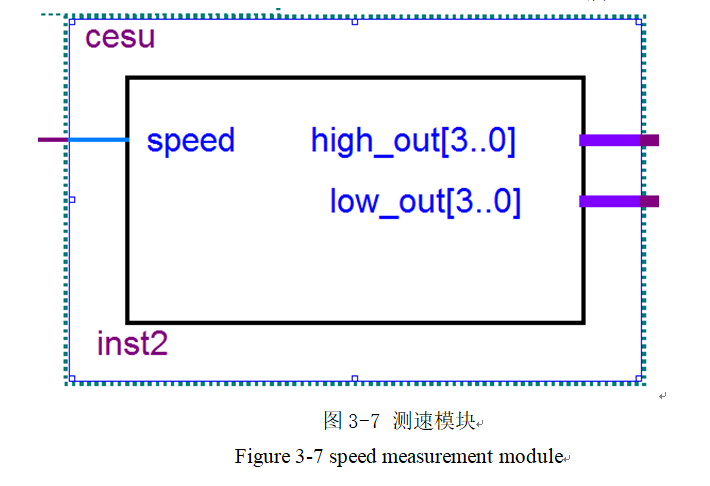Figure 3-7 speed measurement module

library ieee;

use ieee.std_logic_unsigned.all;

use ieee.std_logic_1164.all;

entity cesu is

port(speed:in std_logic;

high_out:out std_logic_vector(3 downto 0);

low_out:out std_logic_vector(3 downto 0)

);

end cesu;

architecture b1 of cesu is

signal jj:std_logic;

begin

process(speed)

variable cnta:std_logic_vector(1 downto 0);

variable gw:std_logic_vector(3 downto 0);

variable sw:std_logic_vector(3 downto 0);

begin

if(speed=‘0’) then

cnta:=cnta+1;

end if;

if(cnta<“11”) then

jj<=‘0’;

elsif(cnta=“11”) then

jj<=‘1’;

end if;

if(jj’event and jj=‘1’) then

if(gw<9) then

gw:=gw+1;

sw:=sw;

elsif(gw=9) then

gw:=“0000”;

sw:=sw+1;

end if;

end if;

high_out<=sw;

low_out<=gw;

end process;

end;

[外链图片转存失败,源站可能有防盗链机制,建议将图片保存下来直接上传(img-nXpsuNJM-1618936139701)(media/93a46dd7242900e496d0457ca900c248.png)]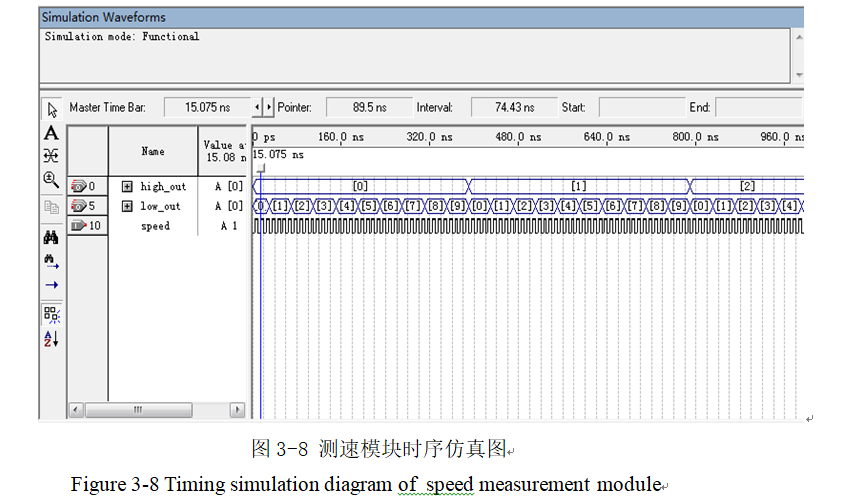Figure 3-8 Timing simulation diagram of speed measurement module

### 3.3.5 数码管显示模块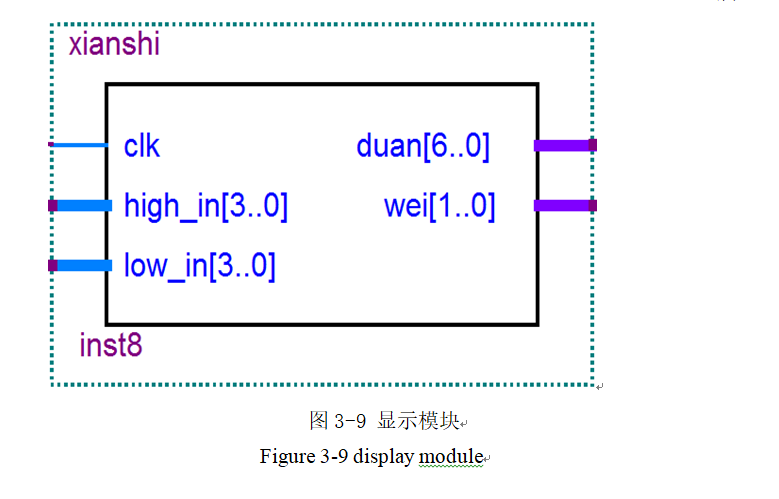Figure 3-9 display module

library ieee;

use ieee.std_logic_1164.all;

use ieee.std_logic_unsigned.all;

entity xianshi is

port(clk:in std_logic;

high_in:in std_logic_vector(3 downto 0);

low_in:in std_logic_vector(3 downto 0);

duan:out std_logic_vector(6 downto 0);

wei:out std_logic_vector(1 downto 0)

);

end xianshi;

architecture b2 of xianshi is

Type states is (s0,s1);

signal st,nst: states:=s0;

signal qq:Std_Logic_vector(3 downto 0);

begin

process(high_in,low_in,st,clk)

begin

case st is

when s0=> qq<=low_in;wei<=“10”;

When s1=> qq<=high_in;wei<=“01”;

when others=> qq<=qq;wei<=“11”;

end case;

CASE qq IS

When “0000”=> duan<=“1000000”;

When “0001”=> duan<=“1111001”;

When “0010”=> duan<=“0100100”;

When “0011”=> duan<=“0110000”;

When “0100”=> duan<=“0011001”;

When “0101”=>duan<=“0010010”;

When “0110”=> duan<=“0000010”;

When “0111”=> duan<=“1111000”;

When “1000”=> duan<=“0000000”;

When “1001” => duan<=“0010000”;

When others => duan<=“1111111”;

end case;

CASE st IS

When s0=> nst<= s1;

When s1=> nst<= s0;

end case;

end process;

reg: PROCESS(clk)–zhukong shixu jincheng

begin

if clk’Event and clk = ‘1’ then st<=nst;

End if;

End PROCESS reg;

end;

### 3.3.6 消抖模块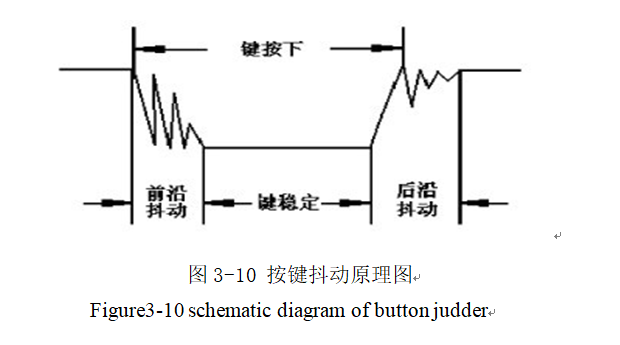Figure3-10 schematic diagram of button judder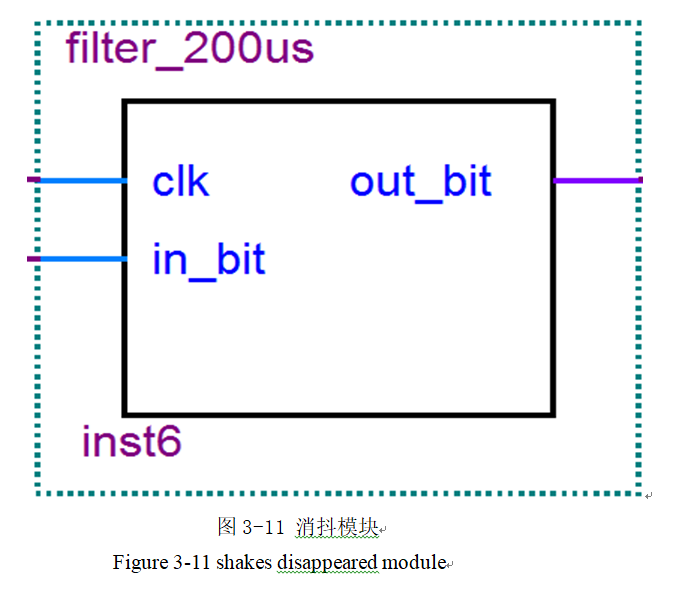Figure 3-11 shakes disappeared module

LIBRARY IEEE;

USE IEEE.STD_LOGIC_1164.ALL;

USE IEEE.STD_LOGIC_Arith.ALL;

USE IEEE.STD_LOGIC_Unsigned.ALL;

ENTITY filter_200us IS

PORT(

clk,in_bit: IN STD_LOGIC;

out_bit: OUT STD_LOGIC

);

END;

ARCHITECTURE one OF filter_200us IS

SIGNAL cnt:STD_LOGIC_VECTOR(19 DOWNTO 0);

SIGNAL out_bit_r:STD_LOGIC;

BEGIN

out_bit<=out_bit_r;

PROCESS(clk)

BEGIN

IF RISING_EDGE(clk) THEN

IF in_bit =‘1’ THEN

cnt <=X"00000";

out_bit_r <=‘1’; ELSE

IF cnt>=480000 THEN out_bit_r<=‘0’; ELSE .

cnt<=cnt+1; out_bit_r<=‘1’;

END IF;

END IF;

END IF;

END PROCESS;

END;

# 参考文献

 潘志浪.基于FPGA的DDS信号源的设计[D].武汉:武汉理工大学信息工程学院，2007.

 周立功,等.EDA实验与实践[M].北京:北京航空航天大学出版社,2007.

 王美妮.基于FPGA的DDS的研究设计与实现[D].大连：大连海事大学，2006.



 潘松 黄继业编著,《EDA技术实用教程》[M],科学技术出版,2005年

陶东娅.

李凡.基于FPGA的全数字无刷直流电机控制系统研究[D].重庆：重庆大学，2010年

王毓银.《数字电路逻辑设计》[M]，高等教育出版社，1999年

田萍,刘新云;用VHDL语言实现数字系统[J];现代电子技术;2004年07期

何西凤;黄念慈;;数字化通用PWM控制器的设计[J];电源技术应用;2006年05期

# 附 录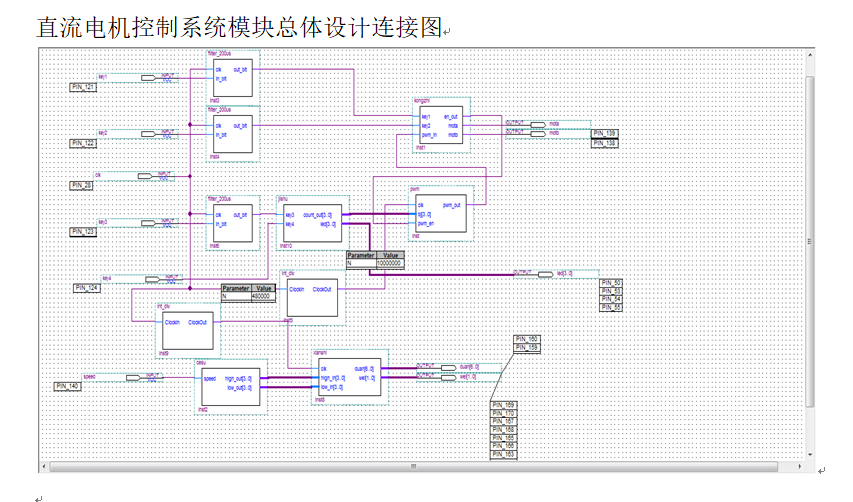# 致 谢

，《基于DDS原理的任意波形信号发生器的设计》[M],现代机械,2000年

 潘松 黄继业编著,《EDA技术实用教程》[M],科学技术出版,2005年

陶东娅.

李凡.基于FPGA的全数字无刷直流电机控制系统研究[D].重庆：重庆大学，2010年

王毓银.《数字电路逻辑设计》[M]，高等教育出版社，1999年

田萍,刘新云;用VHDL语言实现数字系统[J];现代电子技术;2004年07期

何西凤;黄念慈;;数字化通用PWM控制器的设计[J];电源技术应用;2006年05期

# 附 录

[外链图片转存中…(img-xUEdEoQ0-1618936139704)]

# 致 谢

08-23
04-26247511-18
04-15
07-31
07-04798
03-21454
05-03点击重新获取扫码支付余额充值